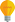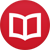# Integral of $x^n$

by Jakub MarianTip: See my list of theMost Common Mistakes in English. It will teach you how to avoid mis­takes with com­mas, pre­pos­i­tions, ir­reg­u­lar verbs, and much more.

How to calculate the indefinite integral of $x^n$? It’s quite simple. We are looking for a function $f(x)$ such that $f’(x) = x^n$. As you surely know, $$(x^m)’ = mx^{m-1}\,.$$ If we choose $m = n+1$, we get the power we want: $$(x^{n+1})’ = (n+1)x^n\,.$$ If we divide both sides by $n+1$ (which is a constant and thus does not influence the derivative), we’ll get the function we want: $$\l(\frac{x^{n+1}}{n+1}\r)’ = x^n\,.$$ Hence we conclude that $$∫ x^n\,dx = \frac{x^{n+1}}{n+1}+c\,.$$ There is one problem, however. The formula above works for any real number (even a fraction or an irrational number) except $n = -1$ (which gives $x^0/0$, which is obviously not right). If you remember your derivatives, you’ll recall that $$\l(\log(x)\r)’ = \frac{1}{x}$$ (where $\log(x)$, sometimes denoted $\ln(x)$, is the natural logarithm), so $∫ x^{-1}\,dx = ∫ \frac{1}{x}\,dx = \log(x) + c$. All in all, we can write: $$∫ x^n\,dx = \begin{cases}\frac{x^{n+1}}{n+1} +c & n ∈ ℝ \setminus \{-1\}\\ \log(x) +c & n = -1 \end{cases}$$

By the way, I have written several educational ebooks. If you get a copy, you can learn new things and support this website at the same time—why don’t you check them out?